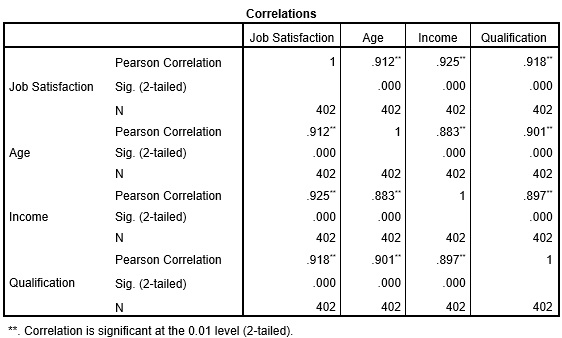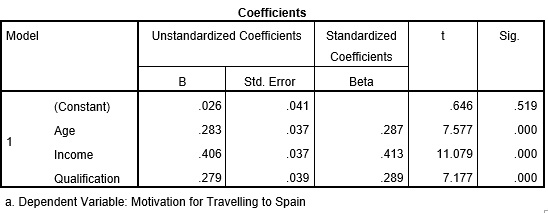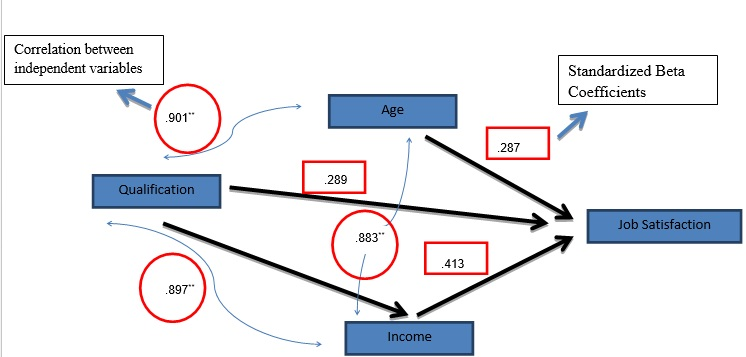# How to conduct path analysis?

By Indra Giri and Priya Chetty on November 5, 2017
Image by Pressfoto on Freepik

Path analysis is a graphical representation of multiple regression models. In this analysis, the graphs represent the relationship between the dependent and independent variables with the help of square and arrows. These graphs show the causation between different variables of a regression model. To conduct a path analysis, simply write the names of variables in square boxes and connect the square boxes with arrows. This will indicate the effect of one on another, similar to regression. Path analysis takes effect in two ways; before and after running the regression. They are also known as ‘input path diagram’ and ‘output path diagram’ respectively. While the input path diagram represents the causal connections between variables while proposing a hypothesis, the output path diagram shows the actual outcome of the test. These outcomes help test the hypothesis propose in input path diagrams.

## Understanding path analysis

In this case study, there are three independent variables; ‘age’, ‘income’ and ‘qualification’. These variables affect the ‘job satisfaction’ of employees. Therefore, ‘job satisfaction’ becomes the dependent variable and the others become the independent variables. The hypotheses for this case are:

• Hypothesis 1: Qualification has a positive impact on job satisfaction.
• Hypothesis 2: Income has a positive impact on job satisfaction.
• Hypothesis 3: Age has a negative impact on job satisfaction.

Firstly, construct the input path diagram to show the causal connections put forth in these hypotheses. The path diagram for the hypotheses appears as under:Figure 1: a Hypothesized input path analysis

The above input diagram displays an intuitive idea of how age, qualification and income affect job satisfaction. Since one of the above hypotheses proposes a negative impact of age on job satisfaction, that line is red. The diagram is simple and assumes all three variables have a direct impact on the dependent variable.

In the above case, input path analysis shows the hypothesized relationship. Furthermore, it only includes the effect of the independent variable on the dependent variables. However, these three independent variables can also have an effect on each other. Therefore, output path diagrams can be constructed after performing correlation and regression analysis. The items undertaken by output path analysis include:

## Correlation coefficients

SPSS software is used for analysing this case. The images below show the correlation between the variables in the model. ‘Sig. (2-tailed)’ indicates the significance of the correlation coefficient. The correlation coefficients are significant if the significance value is less than or equal to 0.05.Results from Pearson correlation analysis conducted in SPSS
Use 5E25A5EE63214 to save 5000 on 15001 - 20000 words standard order of literature survey service.

## Standardized beta coefficients in regression results

A standardized beta coefficient is important for path diagrams because it represents a direct impact of an independent variable on the dependent variable. The regression results have been shown in below two images.Regression results from SPSSRegression results from SPSS

Here, the regression results components taken for output path analysis includes standardized beta coefficients and correlation coefficients. The output path analysis will appear as under:Figure 2: Output path analysis based on correlation coefficients

Furthermore, the below given numbers represent the correlation between independent variables and standardized beta coefficients.Figure 3: Output path analysis based on correlation and regression coefficients

On the basis of output path analysis, the conclusion is that the prediction about the positive and significant impact of income and qualification is true. However, the negative impact of age on job satisfaction is rejected as the regression coefficient of age is positive.

Use 5E25AA6079691 to save 14000 on 32001 - 38000 words standard order of thesis writing service.

## Application of path analysis

Since path analysis is the extension of regression analysis, it is only useful in cases applying regression analysis techniques.

## Software supporting path analysis

Software that supports this analysis with multiple regressions is R, SAS, MATLAB, STATA, and SPSS (AMOS version only).

 How to apply logistic regression in a case? How to perform nonlinear regression?